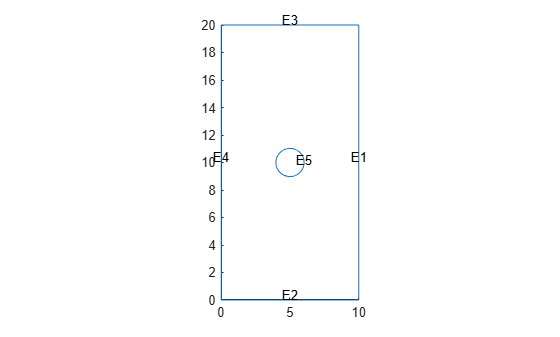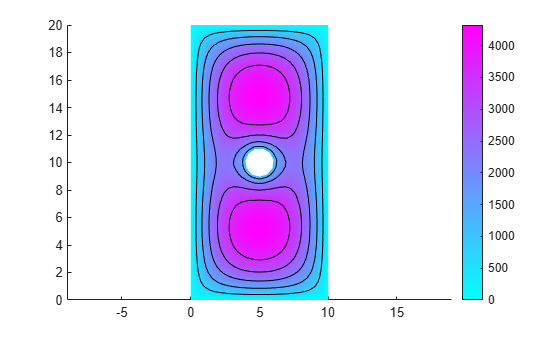# ElectrostaticResults

Electrostatic solution and derived quantities

## Description

An `ElectrostaticResults` object contains the electric potential, electric field, and electric flux density values in a form convenient for plotting and postprocessing.

The electric potential, electric field, and electric flux density are calculated at the nodes of the triangular or tetrahedral mesh generated by `generateMesh`. Electric potential values at the nodes appear in the `ElectricPotential` property. Electric field values at the nodes appear in the `ElectricField` property. Electric flux density at the nodes appear in the `ElectricFluxDensity` property.

To interpolate the electric potential, electric field, and electric flux density to a custom grid, such as the one specified by `meshgrid`, use the `interpolateElectricPotential`, `interpolateElectricField`, and `interpolateElectricFlux` functions.

## Creation

Solve an electrostatic problem using the `solve` function. This function returns a solution as an `ElectrostaticResults` object.

## Properties

expand all

Electric potential values at nodes, returned as a vector.

Data Types: `double`

Electric field values at nodes, returned as an `FEStruct` object. The properties of this object contain the components of the electric field at nodes.

Electric flux density values at nodes, returned as an `FEStruct` object. The properties of this object contain the components of electric flux density at nodes.

Finite element mesh, returned as an FEMesh Properties object. For details, see FEMesh Properties.

## Object Functions

 `interpolateElectricPotential` Interpolate electric potential in electrostatic result at arbitrary spatial locations `interpolateElectricField` Interpolate electric field in electrostatic result at arbitrary spatial locations `interpolateElectricFlux` Interpolate electric flux density in electrostatic result at arbitrary spatial locations

## Examples

collapse all

Solve an electromagnetic problem and find the electric potential and field distribution for a 2-D geometry representing a plate with a hole.

Create an electromagnetic model for electrostatic analysis.

`emagmodel = createpde('electromagnetic','electrostatic');`

Import and plot the geometry representing a plate with a hole.

```importGeometry(emagmodel,'PlateHolePlanar.stl'); pdegplot(emagmodel,'EdgeLabels','on')```Specify the vacuum permittivity in the SI system of units.

`emagmodel.VacuumPermittivity = 8.8541878128E-12;`

Specify the relative permittivity of the material.

`electromagneticProperties(emagmodel,'RelativePermittivity',1);`

Apply the voltage boundary conditions on the edges framing the rectangle and the circle.

```electromagneticBC(emagmodel,'Voltage',0,'Edge',1:4); electromagneticBC(emagmodel,'Voltage',1000,'Edge',5);```

Specify the charge density for the entire geometry.

`electromagneticSource(emagmodel,'ChargeDensity',5E-9);`

Generate the mesh.

`generateMesh(emagmodel);`

Solve the model.

`R = solve(emagmodel)`
```R = ElectrostaticResults with properties: ElectricPotential: [1218x1 double] ElectricField: [1x1 FEStruct] ElectricFluxDensity: [1x1 FEStruct] Mesh: [1x1 FEMesh] ```

Plot the electric potential and field.

```pdeplot(emagmodel,'XYData',R.ElectricPotential, ... 'FlowData',[R.ElectricField.Ex ... R.ElectricField.Ey]) axis equal```Solve an electromagnetic problem and find the electric potential and field distribution for a 3-D geometry representing a plate with a hole.

Create an electromagnetic model for electrostatic analysis.

`emagmodel = createpde('electromagnetic','electrostatic');`

Import and plot the geometry representing a plate with a hole.

```gm = importGeometry(emagmodel,'PlateHoleSolid.stl'); pdegplot(gm,'FaceLabels','on','FaceAlpha',0.3)```Specify the vacuum permittivity in the SI system of units.

`emagmodel.VacuumPermittivity = 8.8541878128E-12;`

Specify the relative permittivity of the material.

`electromagneticProperties(emagmodel,'RelativePermittivity',1);`

Specify the charge density for the entire geometry.

`electromagneticSource(emagmodel,'ChargeDensity',5E-9);`

Apply the voltage boundary conditions on the side faces and the face bordering the hole.

```electromagneticBC(emagmodel,'Voltage',0,'Face',3:6); electromagneticBC(emagmodel,'Voltage',1000,'Face',7);```

Generate the mesh.

`generateMesh(emagmodel);`

Solve the model.

`R = solve(emagmodel)`
```R = ElectrostaticResults with properties: ElectricPotential: [4359x1 double] ElectricField: [1x1 FEStruct] ElectricFluxDensity: [1x1 FEStruct] Mesh: [1x1 FEMesh] ```

Plot the electric potential.

```figure pdeplot3D(emagmodel,'ColorMapData',R.ElectricPotential)```Plot the electric field.

```pdeplot3D(emagmodel,'FlowData',[R.ElectricField.Ex ... R.ElectricField.Ey ... R.ElectricField.Ez])```